: References : Two dimensional anelastic model : 5 Radiation

# 6 Ground surface

The time integration of 1D thermal conduction equation of grand surface (equation (55) in Part I is performed by the Crank-Nicolson scheme. The space differencing is evaluated by the second order centered scheme. The grand temperature and depth are evaluated on the grid point and the heat flux is evaluated on the half grid point. The number of vertical grid point is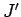and the suffix of the lowest grid point is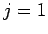. The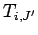is assumed to the surface temperature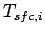. The finite difference 1D thermal conduction equation is represented as follows.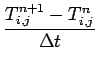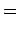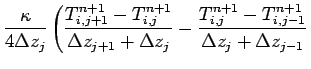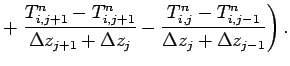(66)

or,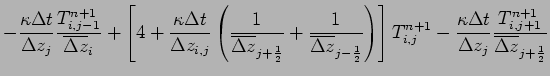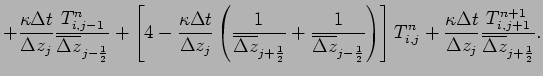(67)

where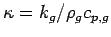. This equation can be represented in matrix form as follows.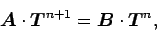(68)

where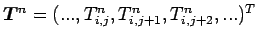. The elements of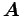,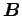are represented as follows.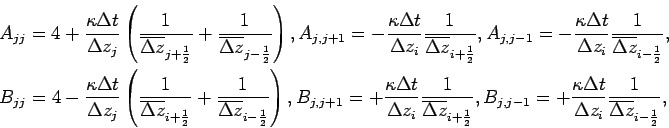Considering the boundary condition of upper and lower boundaries, (68) is modified as follows.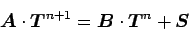(69)

Therefore, the grand temperature is given by the solution of the following matrix equation.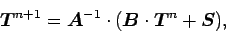(70)

where the elements ofandare modified as follows.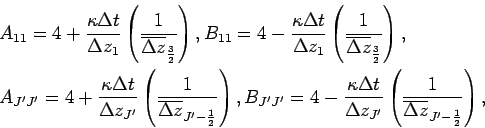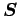is a column vector whose dimension isare represented as follows.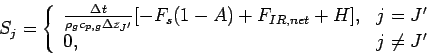: References : Two dimensional anelastic model : 5 Radiation
Odaka Masatsugu $BJ?@.(B19$BG/(B4$B7n(B26$BF|(B